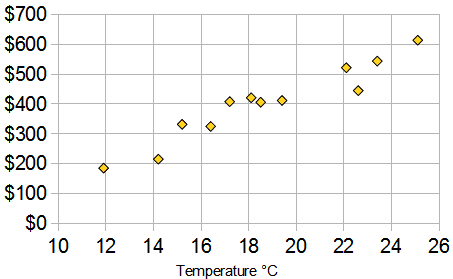# Unit 6: Reading Graphs

Page

#### The graphs are about having data for two variables (x, y) . Typically the two types of data are related in some way.

Below is a Scatter Plot with bivariate data. The two things that we are comparing are Temperature and Ice Cream Sales.

Here we have ice cream sales versus the temperature on that day. The two variables are Ice Cream Sales and Temperature.

###### (If you have only one set of data, such as just Temperature, it is called “Univariate Data, bivariate mean two types of data)In this scatter plot you can see that as tempurate rises so do prices.

https://www.mathsisfun.com/definitions/bivariate-data.html

To find more information in Bivariate Data click here.

### Slope Intercept Form:      y = mx + b

The variable m is the slope, it explains the steepness of a line.

The variable b is the y-intercept, this is where the line crosses or intersects at the y-axis.

http://www.shmoop.com/video/slope-intercept-form/